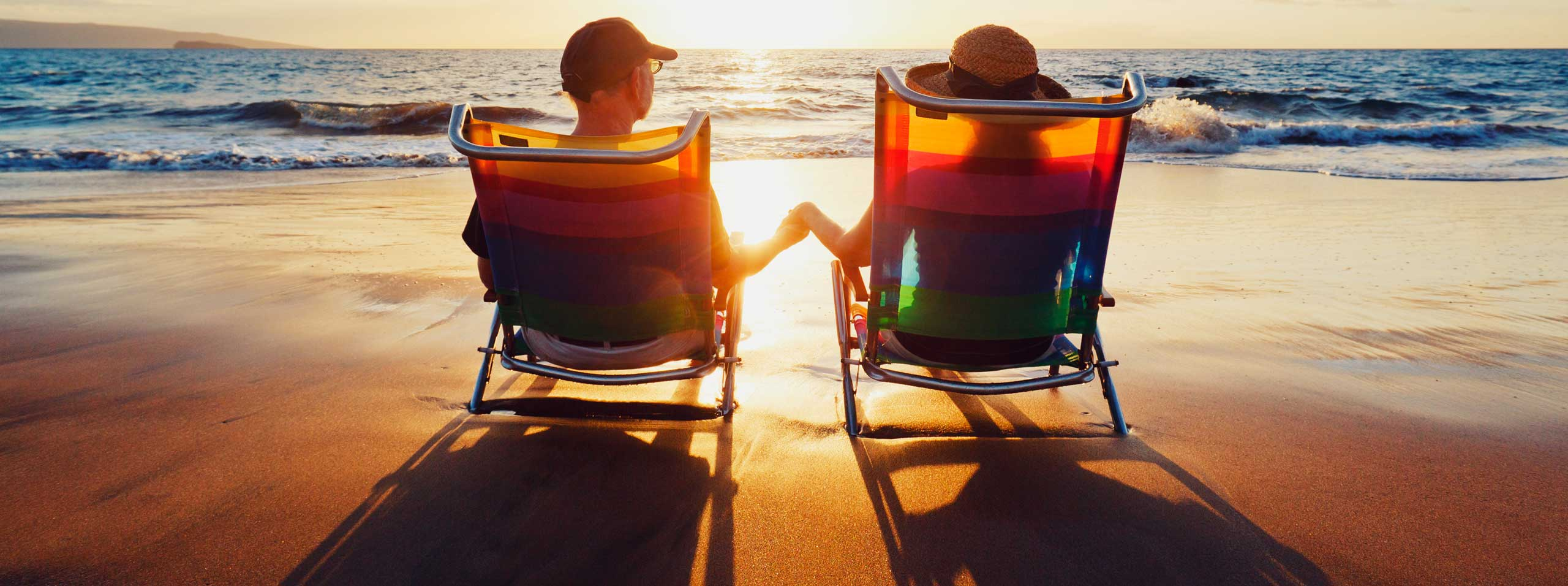# Free GRE Practice Questions with Solutions Sample 1

A set of 10 questions similar to GRE quantitative reasoning questions , with detailed solutions are presented.

## Question 1

A car covered 130 miles using 4 gallons of diesel. What distance would the same car cover, under similar conditions, on 6.7 gallons?
A) 77
B) 260.25
C) 520
D) 217.75
E) 871

## Question 2

If plotted in the same rectangular system of axis, the graphs of f(x) = | |x| - 4 | and g(x) = 2 will have
A) no points of intersection
B) 1 point of intersection
C) 2 points of intersection
D) 3 points of intersection
E) 4 points of intersection

## Question 3

Which of the following is the largest?
A) 125%
B) 1.25
C) 1 + 1/3
D) 4/3
E) 0.0015/0.001

## Question 4

| |- 10 - 19| - 20 | = ?
A) 9
B) 49
C) - 49
D) 19
E) 11

## Question 5

The algebraic expression x / (x + 2) is undefined if x =
A) 0
B) 1
C) 2
D) - 2
E) - 1

## Question 6

(√5 - √7)(√5 + √7) = ?
A) 12
B) 2
C) 24
D) - 24
E) - 2

## Question 7

In the figure below the sides AB and AC of triangle ABC have equal lengths. Find the size of angle ABC..

A) 46�
B) 67�
C) 134�
D) 136�
E) Cannot be calcualated using the given information

## Question 8

If y= 10� in the figure below, what is the value of x?.

A) 20�
B) 30�
C) 10�
D) 5�
E) 15�

## Question 9

By what percent will the volume of a rectangular solid increase if its length, width and height increase by 25% each?

A) 25%
B) 95%
C) 74%
D) 15.6%
E) 50%

## Question 10

What is the average of all prime numbers between 20 and 40?
A) 20
B) 25
C) 30
D) 35
E) 40

## More References and Links to Maths Practice Tests

Free GRE Quantitative for Practice
Free Practice for GAMT Maths tests
Free Compass Maths tests Practice
Free Practice for SAT, ACT Maths tests
Free AP Calculus Questions (AB and BC) with Answers